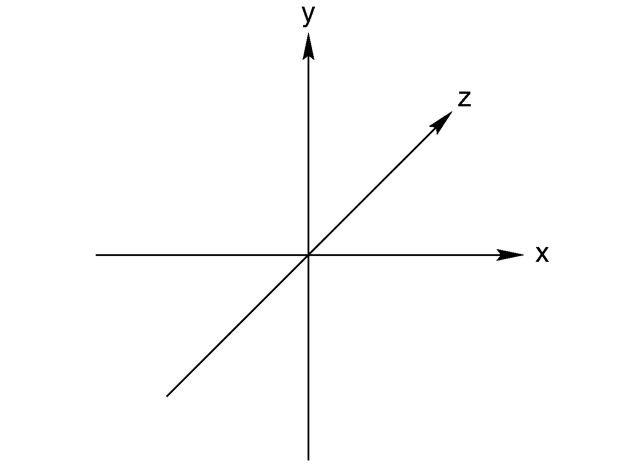User Name Remember Me? Password

 Applied Math Applied Math Forum

 May 13th, 2015, 09:33 AM #1 Senior Member   Joined: Sep 2013 From: Earth Posts: 827 Thanks: 36 Question on the symbol sign. For example, a brick is dropped down a disused well, 50m deep. So I know that $\displaystyle a=9.8$, $\displaystyle s=50$ and $\displaystyle v=0$ So, $\displaystyle s=vt-\frac{1}{2}at^2$ $\displaystyle 50=-\frac{1}{2}(9.8)t^2$ $\displaystyle 50=-4.9t^2$ $\displaystyle t^2=-10.204$ As I know, negative value can't be squared root. But if I ignore the negative sign, I will get the answer which is 3.19 second. Can any explain to me?May 13th, 2015, 10:26 AM #2 Math Team   Joined: Jul 2011 From: Texas Posts: 3,002 Thanks: 1587 $s$ is displacement ... $s=-50 \text{ m}$ Thanks from topsquark and jiasyuenMay 13th, 2015, 10:33 AM #3 Math Team   Joined: May 2013 From: The Astral plane Posts: 2,256 Thanks: 926 Math Focus: Wibbly wobbly timey-wimey stuff. Always always always set up a coordinate system. You won't have as many troubles that way. -DanMay 13th, 2015, 06:38 PM #4 Senior Member   Joined: Sep 2013 From: Earth Posts: 827 Thanks: 36 How to set up a coordinate system ?May 14th, 2015, 12:56 AM #5 Senior Member   Joined: Sep 2013 From: Earth Posts: 827 Thanks: 36 For question b. It asks with what speed does it hit the bottom. I can't solve it.May 14th, 2015, 02:31 AM   #6
Senior Member

Joined: Apr 2014
From: Glasgow

Posts: 2,157
Thanks: 731

Math Focus: Physics, mathematical modelling, numerical and computational solutions
I think you accidentally put $\displaystyle s = ut - \frac{1}{2}at^2$ when you should have put $\displaystyle s = ut + \frac{1}{2}at^2$.

Nevertheless... to avoid problems like this, this is what I like to do...

Draw a diagram of the situation and label the forces. Then, over to one side, place a little arrow (the direction depends on your problem) and put a "+" on it... sort of like the attached image below.

The reason for this? It is a little reminder to make sure you know which direction you are considering to be positive. Therefore, with my arrow there like that, the downward force is positive:

$\displaystyle F = mg$

If the brick was thrown upwards, I would have to use a negative initial velocity.

In your case the brick was just dropped, so you have a downwards (positive) acceleration and the displacement is downwards (i.e. positive)...

$\displaystyle a = 9.8 ms^{-2}$

$\displaystyle s = ut + \frac{1}{2}at^2$
$\displaystyle = 4.9 t^2$

The arrow thing above is similar to axes in 2D or 3D maths problems, where it is common practise to place a little axes by the diagram to show the directions. Something like this:Attached Imagestemp1.jpg (6.4 KB, 2 views)

Last edited by Benit13; May 14th, 2015 at 02:45 AM.May 14th, 2015, 07:02 AM   #7
Senior Member

Joined: Apr 2014
From: Glasgow

Posts: 2,157
Thanks: 731

Math Focus: Physics, mathematical modelling, numerical and computational solutions
Quote:
 Originally Posted by jiasyuenFor question b. It asks with what speed does it hit the bottom. I can't solve it.
Try $\displaystyle v = u + at$May 14th, 2015, 08:32 AM #8 Math Team   Joined: May 2013 From: The Astral plane Posts: 2,256 Thanks: 926 Math Focus: Wibbly wobbly timey-wimey stuff. Setting up a coordinate system. Let's use your problem as an example instead of something more general. You need to choose positive directions. You probably haven't covered the motion equations this way but s, v, and a are all vectors. They have a direction associated with them. All we need to worry about is the positive direction. When dealing with a kinematics problem most people choose + to be up (or when using a horizontal application + to the right.) You need to choose an origin. The motion equations carry information about where you start "counting." You can use the equations no matter what the choice of 0 point is. Most people either choose the origin to be the "place where the problem starts" (ie. where ball was fired upward) or to be the lowest point in the problem. This is hidden by your notation: s is actually $\displaystyle y - y_0$...the origin is assumed in the form of the equations. So for your problem let's use the "usual coordinate system": origin at the place where the brick was dropped, and + upward. Thus the starting point for the y axis is 0, the final point is s = -50, negative because it's below the origin, v = 0 as it's dropped from rest, and a = -g as upward is positive. Thus $\displaystyle s = ut + (1/2)at^2$ becomes $\displaystyle -50 = -(1/2)gt^2$. The signs take care of themselves. -DanTags question, sign, symbolThread ToolsShow Printable VersionEmail this Page Display ModesLinear ModeSwitch to Hybrid ModeSwitch to Threaded ModeSimilar Threads Thread Thread Starter Forum Replies Last Post MadSoulz Applied Math 6 September 20th, 2014 02:42 PM smartcookie Algebra 8 November 14th, 2012 12:43 AM Jamsisos Algebra 2 July 6th, 2012 03:02 AM tsl182forever8 Algebra 6 February 11th, 2012 05:06 PM adrolc Algebra 1 March 3rd, 2009 01:08 PM

 Contact - Home - Forums - Cryptocurrency Forum - Top

Copyright © 2019 My Math Forum. All rights reserved.